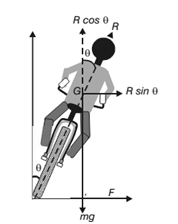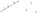# Angled cyclist turn

The cyclist passes through a curve with a radius of 20 m at 25 km/h. How much angle does it have to bend from the vertical inward to the turn?

Result

A =  13.557 °

#### Solution:

$r=20 \ \text{m} \ \\ v=25 \ km/h=25 / 3.6 \ m/s=6.94444 \ m/s \ \\ g=10 \ \text{m/s}^2 \ \\ \ \\ F_o + F_g=F \ \\ F_g=m \cdot \ g \ \\ F_o=m v^2 / r \ \\ \ \\ \tan A=\dfrac{ F_o }{ F_g } \ \\ \tan A=\dfrac{ m v^2 / r }{ m \cdot \ g } \ \\ \tan A=\dfrac{ v^2 }{ r \cdot \ g } \ \\ \ \\ A_{1}=\arctan(\dfrac{ v^2 }{ r \cdot \ g } )=\arctan(\dfrac{ 6.9444^2 }{ 20 \cdot \ 10 } ) \doteq 0.2366 \ \text{rad} \ \\ \ \\ A=A_{1} \rightarrow \ ^\circ =A_{1} \cdot \ \dfrac{ 180 }{ \pi } \ \ ^\circ =13.55675 \ \ ^\circ =13.557 ^\circ =13^\circ 33'24"$Our examples were largely sent or created by pupils and students themselves. Therefore, we would be pleased if you could send us any errors you found, spelling mistakes, or rephasing the example. Thank you!

Leave us a comment of this math problem and its solution (i.e. if it is still somewhat unclear...):

Showing 1 comment:Matematik
A cyclist has to bend slightly towards the center of the circular track in order to make a safe turn without slipping.

Let m be the mass of the cyclist along with the bicycle and v, the velocity. When the cyclist negotiates the curve, he bends inwards from the vertical, by an angle θ. Let R be the reaction of the ground on the cyclist. The reaction R may be resolved into two components:

(i) the component R sin θ, acting towards the center of the curve providing necessary centripetal force for circular motion and
(ii) the component R cos θ, balancing the weight of the cyclist along with the bicycle.

Thus for less bending of the cyclist (i.e for θ to be small), the velocity v should be smaller and radius r should be larger. let h be the elevation of the outer edge of the road above the inner
edge and l be the width of the road then,Tips to related online calculators
Two vectors given by its magnitudes and by included angle can be added by our vector sum calculator.
Do you have a linear equation or system of equations and looking for its solution? Or do you have quadratic equation?
Do you want to convert mass units?
Do you want to convert velocity (speed) units?

## Next similar math problems:

1. Right angled triangle 2LMN is a right angled triangle with vertices at L(1,3), M(3,5) and N(6,n). Given angle LMN is 90° find n
2. Right triangle from axesA line segment has its ends on the coordinate axes and forms with them a triangle of area equal to 36 square units. The segment passes through the point ( 5,2). What is the slope of the line segment?
3. Find the 10Find the value of t if 2tx+5y-6=0 and 5x-4y+8=0 are perpendicular, parallel, what angle does each of the lines make with the x-axis, find the angle between the lines?
4. CuboidsTwo separate cuboids with different orientation in space. Determine the angle between them, knowing the direction cosine matrix for each separate cuboid. u1=(0.62955056, 0.094432584, 0.77119944) u2=(0.14484653, 0.9208101, 0.36211633)
5. Three points 2The three points A(3, 8), B(6, 2) and C(10, 2). The point D is such that the line DA is perpendicular to AB and DC is parallel to AB. Calculate the coordinates of D.
6. Angle of the body diagonalsUsing vector dot product calculate the angle of the body diagonals of the cube.
7. Two peopleTwo straight lines cross at right angles. Two people start simultaneously at the point of intersection. John walking at the rate of 4 kph in one road, Jenelyn walking at the rate of 8 kph on the other road. How long will it take for them to be 20√5 km apa
8. Angle between vectorsFind the angle between the given vectors to the nearest tenth of a degree. u = (-22, 11) and v = (16, 20)
9. LineStraight line passing through points A [-3; 22] and B [33; -2]. Determine the total number of points of the line which both coordinates are positive integers.
10. Find the 5Find the equation with center at (1,20) which touches the line 8x+5y-19=0
11. Vector equationLet’s v = (1, 2, 1), u = (0, -1, 3) and w = (1, 0, 7) . Solve the vector equation c1 v + c2 u + c3 w = 0 for variables c1 c2, c3 and decide weather v, u and w are linear dependent or independent
12. Coordinates of a centroindLet’s A = [3, 2, 0], B = [1, -2, 4] and C = [1, 1, 1] be 3 points in space. Calculate the coordinates of the centroid of △ABC (the intersection of the medians).
13. Parametric formCalculate the distance of point A [2,1] from the line p: X = -1 + 3 t Y = 5-4 t Line p has a parametric form of the line equation. ..
14. Set of coordinatesConsider the following ordered pairs that represent a relation. {(–4, –7), (0, 6), (5, –3), (5, 2)} What can be concluded of the domain and range for this relation?
15. Coordinates of square verticesThe ABCD square has the center S [−3, −2] and the vertex A [1, −3]. Find the coordinates of the other vertices of the square.
16. Points collinearShow that the point A(-1,3), B(3,2), C(11,0) are col-linear.
17. Slope formFind the equation of a line given the point X(8, 1) and slope -2.8. Arrange your answer in the form y = ax + b, where a, b are the constants.